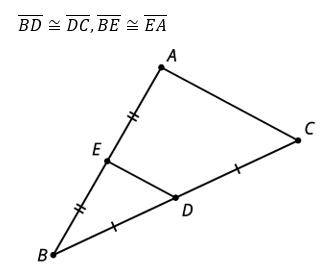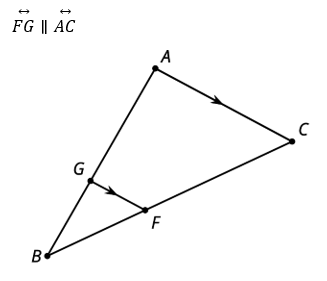# G.3.5 Lesson Summary

Let's examine a segment whose endpoints are the midpoints of 2 sides of the triangle. If D is the midpoint of segment BC and E is the midpoint of segment BA, then what can we say about ED and triangle ABC? Segment ED is parallel to the third side of the triangle and half the length of the third side of the triangle. For example, if AC = 10, then ED = 5. This happens because the entire triangle EBD is a dilation of triangle ABC with a scale factor of 1/2.In triangle ABC, segment FG divides segments AB and CB proportionally. In other words, BG/GA = BF/FC. Again, there is a dilation that takes triangle ABC to triangle GBF, so FG is parallel to AC and we can calculate its length using the same scale factor.